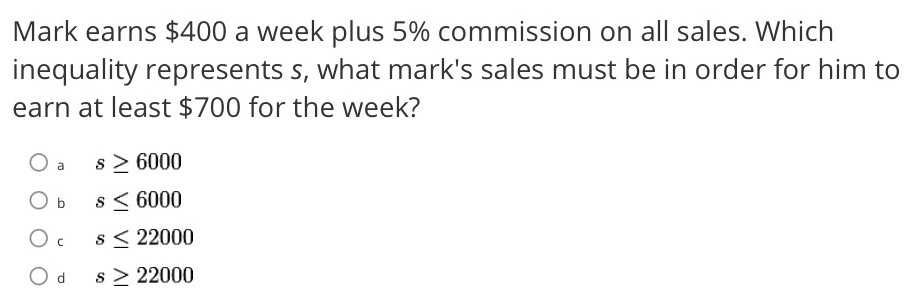### Still have math questions?

Algebra
QuestionMark earns $$\ 400$$ a week plus $$5 \%$$ commission on all sales. Which inequality represents s, what mark's sales must be in order for him to earn at least $$\ 700$$ for the week?

$${ a. } s \geq 6000$$

$${ b. } s \leq 6000$$

$${ c. } s \leq 22000$$

$${ d. } s \geq 22000$$

$$400 + 5\% \ \text{of}\ s \ge 700\\\qquad \qquad \frac{5}{100}s \ge300\\\qquad \qquad \quad s \ge 6000$$